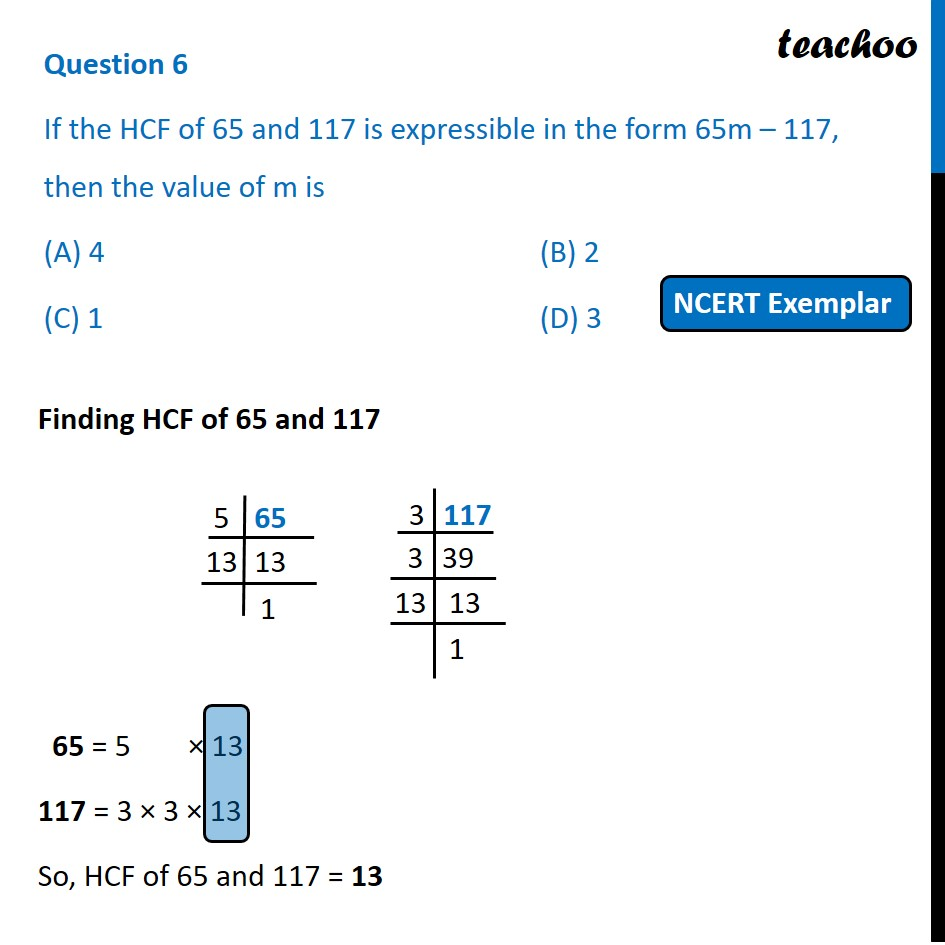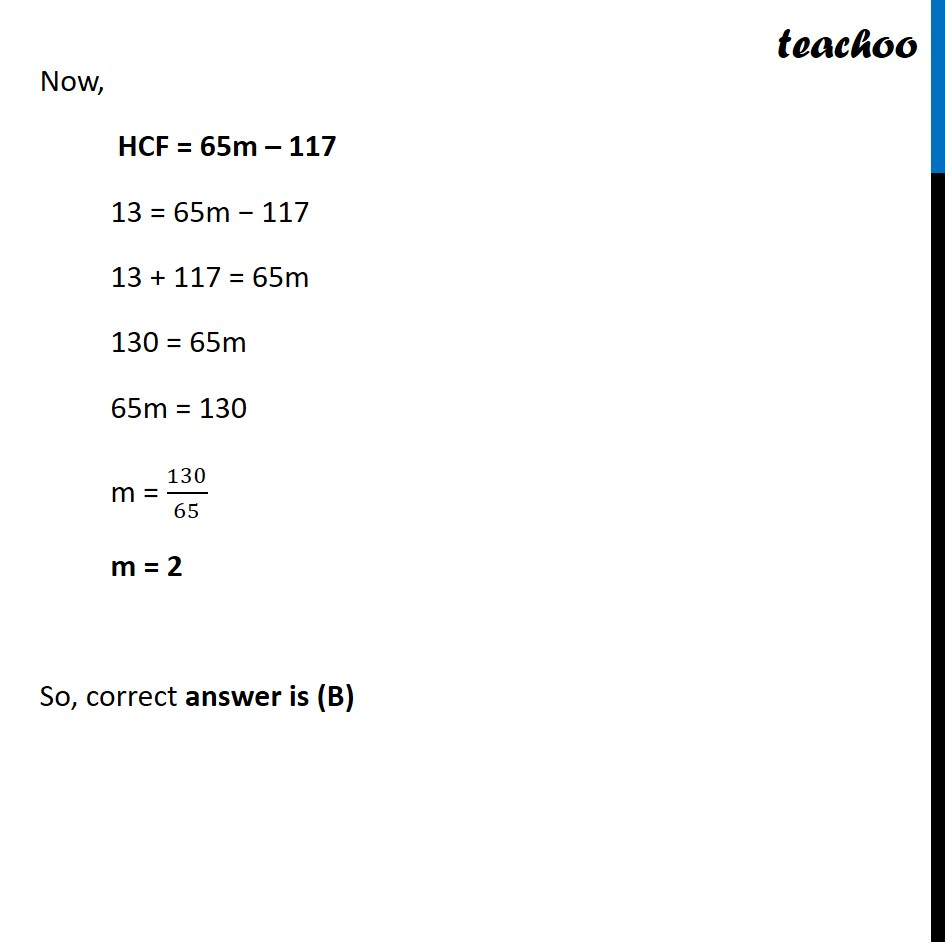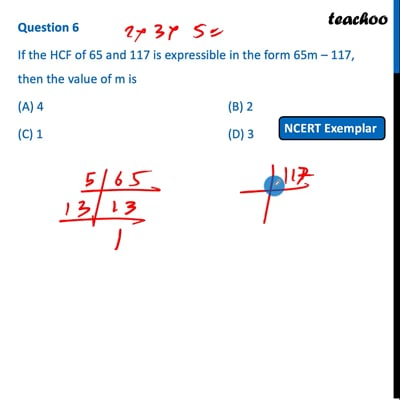MCQs from NCERT Exemplar

Chapter 1 Class 10 Real Numbers
Serial order wise

## (C) 1   (D) 3This video is only available for Teachoo black users

Introducing your new favourite teacher - Teachoo Black, at only ₹83 per month

### Transcript

Question 6 If the HCF of 65 and 117 is expressible in the form 65m – 117, then the value of m is (A) 4 (B) 2 (C) 1 (D) 3 Finding HCF of 65 and 117 65 = 5 × 13 117 = 3 × 3 × 13 So, HCF of 65 and 117 = 13 Now, HCF = 65m – 117 13 = 65m − 117 13 + 117 = 65m 130 = 65m 65m = 130 m = 130/65 m = 2 So, correct answer is (B)